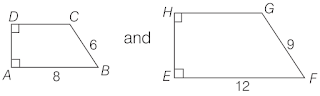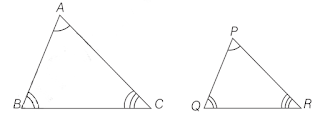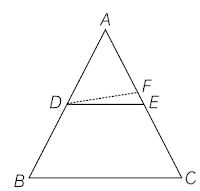## Study Materials and Revision Notes for Ch 6 Triangles Class 10th Mathematics

Triangles

Similarity: Two geometrical figures are said to be similar figures, if they have same shape but not necessarily the same size.
Or
A shape is said to be similar to other, if the ratio of their corresponding sides is equal and the corresponding angles are equal.

• Two polygons having the same number of sides are similar, if:
(i) all the corresponding angles are equal and
(ii) all the corresponding sides are in the same ratio or proportion.If only one condition from (i) and (ii) is true for two polygons, then they cannot be similar.

• The ratio that compares the measurements of two similar shapes, is called the scale factor or representative fraction. It is equal to the ratio of corresponding sides of two figures.
We can use the ratio of corresponding sides to find unknown sides of similar shapes.

• Two triangles are said to be similar triangles, if their corresponding angles are equal and their corresponding sides are proportional (i.e., the ratios between the lengths of corresponding sides are equal). For example: If in ∆ABC and ∆PQR∠A = ∠P, ∠B = ∠Q, ∠C = ∠R and,
AB/PQ = BC/QR = AC/PR
The, △ABC∼△PQR
where, symbol ∼ is read as, ‘is similar to’.
Conversely
If △ABC is similar to △PQR, then
∠A = ∠P, ∠B = ∠Q, ∠C = ∠R and,
AB/PQ = BC/QR = AC/PR
Note: The ratio of any two corresponding sides in two equiangular triangles is always the same.

• If a line is drawn parallel to one side of a triangle to intersect the other two sides in distinct points, then other two sides are divided in the same ratio.
This theorem is known as Basic Proportionality Theorem (BPT) or Thales theorem.

• If a line divides any two sides of a triangle in the same ratio, then the line must be parallel to the third side (converse of basic proportionality theorem).• Let us consider two parallel lines l and m and draw one transversal line t, which intersect l and m at P and Q. Then,(i) Corresponding Angles
The angles on the same side of a transversal line are known as the corresponding angles, if both lie either above the two lines or below the two lines. i.e.,
(a) ∠1and ∠5
(b) ∠2 and ∠6
(c) ∠4 and ∠8
(d) ∠3 and ∠7

(ii) Alternate Interior Angles
The following pairs of angles are called pairs of alternate interior angles
(a) ∠3 and ∠5
(b) ∠2and ∠8

(iii) Consecutive Interior Angles
The pairs of interior angles on same side of the transversal line are called pair of consecutive interior angles.
(a) ∠2 and ∠5
(b) ∠3 and ∠8

(iv) Alternate Exterior Angles
The following pair of angles are called alternate exterior angles
(a) ∠1and ∠7
(b) ∠4and∠6

(v) Vertically Opposite Angles
The following pair of angles are called vertically opposite angles.
(a) ∠1and ∠3
(b) ∠2and ∠4
(c) ∠5 and ∠7
(d) ∠6and ∠8

• The line segment joining the mid-points of any two sides of a triangle is parallel to the third side. (Mid-point theorem)

• The internal bisector of an angle of a triangle divides the opposite side internally in the ratio of the sides containing the angle. (Angle bisector theorem)

• AAA Similarity
(i) In two triangles, if corresponding angles are equal, then their corresponding sides are in the same ratio i.e., they are proportional and hence the two triangles are similar.

(ii) If two angles of one triangle are respectively equal to two angles of another triangle, then the two triangles are similar (because by the angle sum property of a triangle, their third angle will also be equal) and it is called AAA similarity.

• SSS Similarity
In two triangles, if sides of one triangle are proportional to (i.e., in the same ratio as) the sides of the other triangle, then their corresponding angles are equal and hence the two triangles are similar.

• SAS Similarity
If one angle of a triangle is equal to one angle of the other triangle and the sides including these angles are proportional, then the two triangles are similar.

• The ratio of the areas of two similar triangles is equal to the square of the ratio of their corresponding sides.
If ∆ABC and ∆PQR are similar, then
ar (∆ABC)/ar (∆PQR) = (AB/PQ)2 = (AC/PR)2 =(BC/QR)2

• (i) In a right triangle, the square of the hypotenuse is equal to the sum of the squares of the other two sides. (Pythagoras theorem)
(ii) In a triangle, if square of one side is equal to the sum of the squares of the other two sides, then the angle opposite to first side is a right angle. (Converse of Pythagoras theorem)